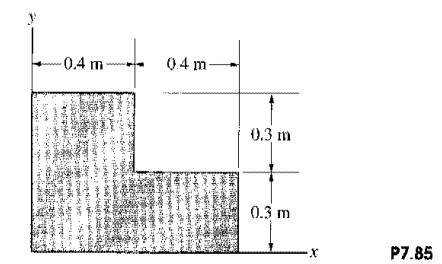### Create an Account

Home / Questions / The mass of the homogeneous thin plate is 36 kg Determine its mass moment of inertia about...

# The mass of the homogeneous thin plate is 36 kg Determine its mass moment of inertia about the x axis 2 Determine the mass moment of inertia of the plate in Problem 785 about the z axis

The mass of the homogeneous, thin plate is 36 kg. Determine its mass moment of inertia about the x axis.2. Determine the mass moment of inertia of the plate in Problem 7.85 about the z axis.

Problem 7.85

The mass of the homogeneous, thin plate is 36 kg. Determine its mass moment of inertia about the x axis.Jun 15 2020 View more View LessSubscribe To Get Solution